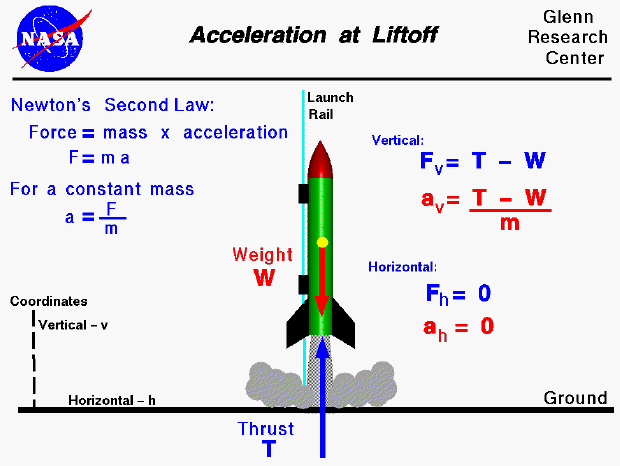The forces on a model rocket change dramatically in both magnitude and direction throughout a typical flight. This figure shows the acceleration on a rocket just following liftoff. The acceleration is produced in response to Newton's first law of motion. A net external force is being applied to the rocket. The only forces present are the thrust (T) and the weight (W).The weight is always directed toward the center of the earth; the direction of the thrust is along the rocket axis. For a vertical launch, the thrust is directly opposite the weight and the net vertical force (Fv) is the thrust minus the weight (Fv = T - W). There is no horizontal force present for a vertical launch. The acceleration of the rocket can be determined by Newton's Second Law of Motion. For a constant mass, this law can be written as force (F) equals mass (m) times acceleration (a); (F = m * a). We can solve for the acceleration using algebra ( a = F / m). Then the vertical acceleration (av) of the rocket is given by the equation ( av = [T - W] / m ).

Since the aerodynamic forces depend on the square of the velocity, and the velocity is low during liftoff, the magnitude of the aerodynamic forces is very small. That is why there is no horizontal component of the acceleration for this vertical launch configuration.

For a model rocket, the weight of the propellant is small relative to the weight of the entire vehicle. So, in determining the acceleration we can assume that the weight remains nearly constant. This is different from a full scale rocket in which the propellants represent a large portion of the weight of the vehicle. Changes in the weight (mass) of the propellant must then be included in any determination of velocity or acceleration during the flight. The equations shown on this slide would not be valid for a full scale rocket because of the change in mass. For a model rocket, the thrust changes greatly with time due to the design of the solid rocket engine. We can use the equations on this slide to determine how the acceleration changes with time for a model rocket.